QUESTION #388

# Is there a precise formula giving the increase in mass due an increase in velocity as described by relativity?

Before I answer your question, I would like to start by giving a more explicit version of Einstein's famous equation, E = mc2. It is commonly written as follows: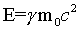where E is the energy of the object in question, m0 is its rest mass, c is the speed of light, and gamma (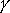) is a numerical factor that depends on velocity. The expression for gamma is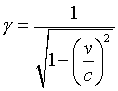where v is the object's velocity. At very small velocities, gamma is approximately equal to one. At velocities near the speed of light, gamma becomes larger and larger (try plugging in some numbers and see). Already you can see why a massive particle can never travel at the speed of light: it would take an infinite amount of energy, and no engine in the universe can provide that sort of power!

Now I will answer your question. Einstein interpreted the combination gamma * m0 to be the mass of an object in motion. This is why the equation is usually quoted simply as E = mc2. The equation relating mass to velocity is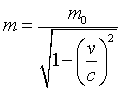Mass, like gamma, grows with velocity. As the object approaches the speed of light, the mass approaches infinity!

I should give a word of caution: many physicists simply call m0 the mass, dropping the distinction between the rest mass and Einstein's velocity-dependent mass. So be careful to note which definition is being used!

This relationship can probably be found in most introductory college physics texts. A good book for more details, at a slightly more technical level, is Ray D'Inverno's book Introducing Einstein's Relativity.

A slightly more technical diversion:

It is interesting to look at the behavior of gamma for small velocities. This can be done using a technique from calculus called Taylor expansion. Consult any calculus text if you want to see exactly what it is and how it's done. If we Taylor expand gamma around v = 0, we get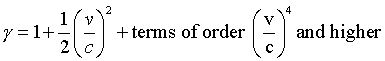For small velocities, the terms of order 4 and greater are extremely tiny compared to the 2nd order term, so we can neglect those all together. Plugging this expression back into Einstein's equation gives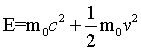The first term is just the rest mass of the object. The second term you may recognize to be the kinetic energy of classical Newtonian physics. Thus Einstein's equation reduces to Newton's equations at small velocities. It took so long to discover that Newton's laws weren't quite correct because the velocities we encounter every day are so much smaller than the speed of light. Thanks to Einstein, we can now study physics at any speed!